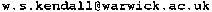# Symbolic Ito Calculus

Symbolic Itô Calculus is the name for my implementation of Itô calculus (stochastic calculus for continuous semimartingales)in various computer algebra packages. See below for various Warwick Statistics Department preprints describing aspects of the work. If you want to get hold of the software then there are various possibilities depending on what is your favourite computer algebra package.

REDUCE: documentation is to be found in Preprint 217 below (Preprint 223 is a shortened version). Alternatively you can download the REDUCE source in the following gzipped tarfile.This work was funded by the SERC under grants GR/E 39891 and GR/E 82538,and also supported by the European Union under a twinning grant.

Mathematica: get hold of a copy of Hal Varian's book "Economic andFinancial Modeling with Mathematica", Springer-Verlag, 1993. On pages 214-238 is my article "Itovsn3: doing stochastic calculus with Mathematica", and the floppy disk provided with the book includes the Mathematica version of symbolic Itô calculus. Preprint 238 below is the preprint form of the article, including listings as an extra item but no disk! Alternatively you can download Itovsn3.m (the source)and various examples in the following gzipped tarfile. This is an updated version which was to be published on the CD-ROM for Mathematics 1999 (which unfortunately never achieved publication): the text describing this is Preprint 333. All this work was supported by the European Union under the HCM network grant ERB CHRX CT94 0449 GCASP(Geometry and Computer Algebra in Statistics and Probability).

AXIOM: I have produced an AXIOM implementation of symbolic Itô calculus (see preprint 327) and Preprint 328 below describes the implementation, including AXIOM listings using the NuWeb implementation of literate programming. Alternatively you can download the AXIOM source in the following gzipped tarfile. This work was funded by EPSRC grant GR/K71677: the summary of the final report for this grant is to be found here.

The other preprints describe various applications; look at them to get an idea of the possibilities. You can read abstracts of my other papers (and some links to downloadable preprints) here. Preprint 247 is the text of a computer-illustrated talk I can give about Brownian motion, which in part provides a brief and elementary introduction to symbolic Itô calculus. Contact me atif you want to find out more about this.

161: The Euclidean diffusion of shape [Abstract].

202: A remark on the proof of Itô's formula for C2 functions of continuous semimartingales [Abstract].

203: Computer algebra and stochastic calculus [Abstract].

217: Symbolic Itô calculus: an introduction [Abstract].

222: (with O.E. Barndorff-Nielsen and P.E. Jupp) Stochastic calculus, statistical asymptotics, Taylor strings and phyla [Abstract].

223: Symbolic Itô calculus: an overview [Abstract].

236: Computer algebra in probability and statistics [Abstract].

237: Computer algebra and yoke geometry I: When is an expression a tensor?[Abstract]

238: Itovsn3: doing stochastic calculus with Mathematica[Abstract].

247: Brownian motion and computer algebra (Text of talk to BAAS ScienceFestival '92, Southampton Wednesday 26 August 1992, with screenshots of illustrative animations) [Abstract].

261: (with G. Ben Arous and M. Cranston) Coupling constructions for hypoelliptic diffusions: Two examples [Abstract].

296: A diffusion model for Bookstein triangle shape [Abstract]:[Gzipped Postscript Paper: 67k].

301: Computer algebra: an article for the Wiley Encyclopaedia of Biostatistics.

327: Symbolic Itô calculus in AXIOM: an ongoing story. [Abstract]:[Gzipped Postscript Paper: 112k] (describesthe new AXIOM implementation).

328: Itovsn3 in AXIOM: modules, algebras, and stochastic differentials. [Abstract]: [GzippedPostscript Paper: 152k] (presents details of the new AXIOM implementation).

331: (with K. Burdzy): Efficient Markovian couplings: examples and counterexamples. [Abstract]: [GzippedPostscript Paper: 224k] (includes incidental application to reflected Brownian motion in a wedge).

333: Stochastic calculus in Mathematica: software and examples.[Abstract] (a slightly updated implementation of the Mathematica version as described in Preprint 238, with a number of extra applications in Mathematica notebook form).

If you want copies of any of these reports then please mail or fax your requests to the departmental secretary.